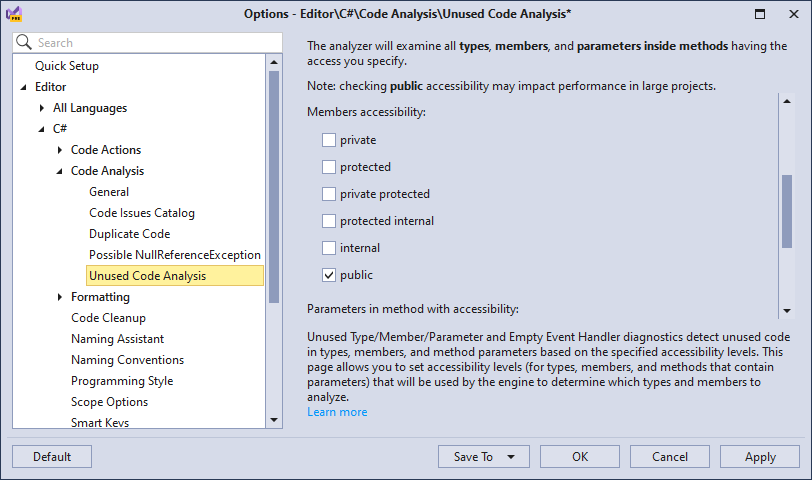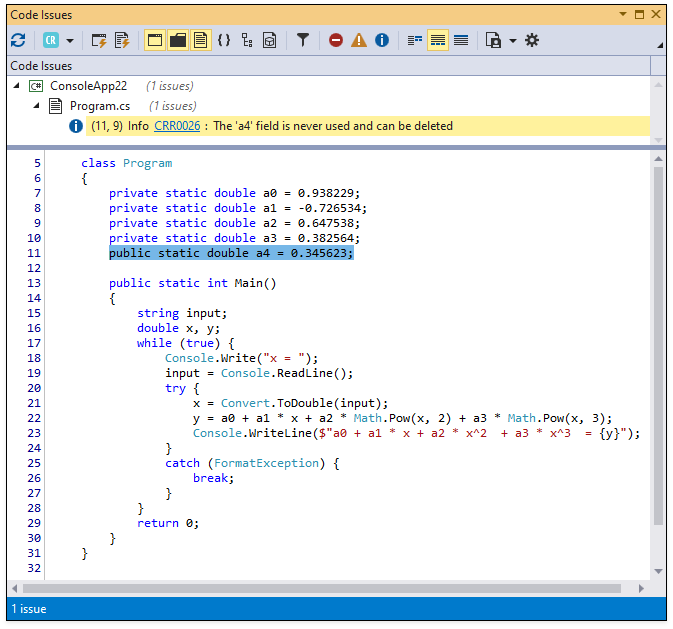# CRR0026 - Unused member

This analyzer detects unused type members. Specify accessibility levels for these members in the Editor | C# (Visual Basic) | Code Analysis | Unused Code Analysis options page. For example, set the public option and uncheck other options to allow CodeRush to detect unused public members only.The following example demonstrates how the analyzer detects an unused public member:Tip

Use the Highlight unused code in editor checkbox on the Editor | C# (Visual Basic) | Code Analysis | General option page to enable or disable the highlighting of unused members in the code editor.

To fix this issue, perform one of the following actions:

1. Remove the public member declaration or add it to your program:

``````class Program {
private static double a0 = 0.938229;
private static double a1 = -0.726534;
private static double a2 = 0.647538;
private static double a3 = 0.382564;

public static int Main() {
string input;
double x, y;
while (true) {
Console.Write("x = ");
try {
x = Convert.ToDouble(input);
y = a0 + a1 * x + a2 * Math.Pow(x, 2) + a3 * Math.Pow(x, 3);
Console.WriteLine(\$"a0 + a1 * x + a2 * x^2  + a3 * x^3 = {y}");
}
catch (FormatException) {
break;
}
}
return 0;
}
}
``````
2. Call the Remove Unused Member refactoring to remove an unused public member.

3. Run Code Cleanup with the Remove unused members rule to remove unused private members.

See the following example for details: How to: Remove Unused Members from Code.